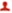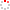NSSC OpenIR  > 微波遥感部
 Random Similarity-Based EntropyAlpha Classification of PolSAR Data Li, Dong; Zhang, YunhuaDepartment 微波遥感部 Source Publication IEEE Journal of Selected Topics in Applied Earth Observations and Remote Sensing2017 ISSN 1939-1404 Language 英语 Abstract A fast and competent alternative to the widely used CloudePottier entropyalpha ($H/ \alpha$) classification is developed for the rapid response application of polarimetric synthetic aperture radar (PolSAR) data. Random similarity which measures both the scattering similarity and randomness of polarimetric scatterers is used to enable an $H/ \alpha$ -like classification in terms of two key parameters, i.e., the similarity-based angle $\alpha _{s}$ and entropy $H_{s}$, as the alternatives to the CloudePottier angle $\alpha$ and entropy H, respectively. Parameters $\alpha _{s}$ and $H_{s}$ maintain the same physical information as parameters $\alpha$ and H, so the existing knowledge regarding $\alpha$ and H can be naturally extended to them. Angle $\alpha _{s}$ measures scattering mechanism and is ranged within the same interval 0, 90 as $\alpha$ while entropy $H_{s}$ measures scattering randomness which is also a logarithm within the interval 0, 1 similar to H. The pixelwise eigendecomposition in the calculation of $\alpha$ and H is avoided for $\alpha _{s}$ and $H_{s}$, and the resulted efficiency improvement is, thus, considerable. By rigorously modeling the $\alpha _{s}\hbox{--}\alpha$ and the $H_{s}\hbox{--}H$ relationship to illustrate the competence of the $H_{s}\hbox{--}\alpha _{s}$ combination in discrimination of target and to identify the searching ranges for the boundary determination, an $H_{s}/ \alpha _{s}$ classification is then devised with the boundaries of the eight effective classes being determined by an optimization to minimize the misclassification and further integrated on different PolSAR images to remove the possible bias from dataset for general applicability. Comparative experiment on both space-borne and airborne PolSAR datasets with $H/ \alpha$ indicates that $H_{s}/ \alpha _{s}$ can achieve very consistent roll-invariant target discrimination as $H/ \alpha$ (overall accuracy 95, kappa coefficient 0.95) but with averagely 150 times higher efficiency although the LAPACK-based eigenanalysis tool has been used to accelerate the eigendecomposition for $H/ \alpha$. Preliminary result from the adaptive model-based classification reveals that the $H_{s}$-involved boundaries in $H_{s}/ \alpha _{s}$ are independent of a particular PolSAR dataset. IEEE Indexed By EI Document Type 期刊论文 Identifier http://ir.nssc.ac.cn/handle/122/6130 Collection 微波遥感部 Recommended CitationGB/T 7714 Li, Dong,Zhang, Yunhua. Random Similarity-Based EntropyAlpha Classification of PolSAR Data[J]. IEEE Journal of Selected Topics in Applied Earth Observations and Remote Sensing,2017. APA Li, Dong,&Zhang, Yunhua.(2017).Random Similarity-Based EntropyAlpha Classification of PolSAR Data.IEEE Journal of Selected Topics in Applied Earth Observations and Remote Sensing. MLA Li, Dong,et al."Random Similarity-Based EntropyAlpha Classification of PolSAR Data".IEEE Journal of Selected Topics in Applied Earth Observations and Remote Sensing (2017).
 Files in This Item: File Name/Size DocType Version Access License 201708047313.pdf（1084KB） 期刊论文 作者接受稿 开放获取 CC BY-NC-SA Application Full Text
 Related Services Recommend this item Bookmark Usage statistics Export to Endnote Google Scholar Similar articles in Google Scholar [Li, Dong]'s Articles [Zhang, Yunhua]'s Articles Baidu academic Similar articles in Baidu academic [Li, Dong]'s Articles [Zhang, Yunhua]'s Articles Bing Scholar Similar articles in Bing Scholar [Li, Dong]'s Articles [Zhang, Yunhua]'s Articles Terms of Use No data! Social Bookmark/Share